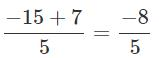Courses

# Test: Comparing Quantities - 3

## 20 Questions MCQ Test Mathematics (Maths) Class 7 | Test: Comparing Quantities - 3

Description
This mock test of Test: Comparing Quantities - 3 for Class 7 helps you for every Class 7 entrance exam. This contains 20 Multiple Choice Questions for Class 7 Test: Comparing Quantities - 3 (mcq) to study with solutions a complete question bank. The solved questions answers in this Test: Comparing Quantities - 3 quiz give you a good mix of easy questions and tough questions. Class 7 students definitely take this Test: Comparing Quantities - 3 exercise for a better result in the exam. You can find other Test: Comparing Quantities - 3 extra questions, long questions & short questions for Class 7 on EduRev as well by searching above.
QUESTION: 1

###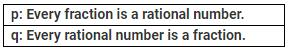Which of the following is correct?

Solution:

Let us consider a fraction 3/5 and a rational number = −4/7 By definition, 3/5 is a rational number.
So, p is true. By definition, −4/7 is not a fraction, since, −4  is not a natural number. So, q is false.

QUESTION: 2

Solution:
QUESTION: 3

### p: All integers are rational numbers. q: Every rational number is an integer. Which of the following statements is correct?

Solution:

Since, every integer having a denominator 1 can be expressed in P/q  form, p is true.
Since, a rational number with denominator other than 1 is not an integer (e.g., 3/5 ), q is false.

QUESTION: 4

What type of a number is −3/0?

Solution:

Since, denominator is 0, it is not a rational number.

QUESTION: 5

Which among the following is a rational number equivalent to 5/3?

Solution:
QUESTION: 6

What type of a numerator does 0/7 have?

Solution:
QUESTION: 7

Which of the following statements is true?

Solution:
QUESTION: 8

If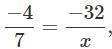what is the value of x?

Solution:
QUESTION: 9

Which is the greatest?

Solution:

Since we know , greater the denominator smaller is the number, but since all numbers are negative, the number nearer to zero will be greatest ,so -5/17 is the greatest number.

QUESTION: 10

Which is the correct descending order of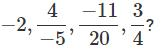Solution:

L.C.M. of 5, 4 and 20 is 20.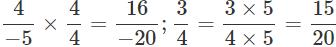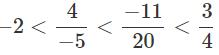or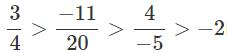is the required descending order. Rewrite equivalent fractions of the given fractions and then compare then. Arrange them in descending order.

QUESTION: 11

What is the average of the two middle rational numbers if 4/7,1/3,2/5 and 5/9 are arranged in ascending order?

Solution:

The ascending order of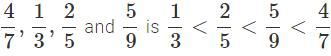The two middle numbers are 2/5 and 5/9
∴  Average
=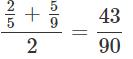QUESTION: 12

What is the percentage of the least number in the greatest number of 3/5,9/5,1/5  and 75 ?

Solution:

The given numbers can be arranged in ascending order as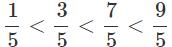The greatest number = 9/5; The least number = 1/5
We have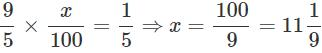QUESTION: 13

What is the difference between the greatest and least numbers of 5/9,1/9 and 11/9 ?

Solution:

The ascending order of given numbers is 1/9,5/9,11/9.
∴  Required difference 11/9−1/9 = 10/9

QUESTION: 14

Which of the following pairs represent the same rational number?

Solution:

−24/15  is the equivalent rational number of −8/5.

QUESTION: 15

P: The quotient of two integers is always a rational number.
Q : 1/0 is not rational. Which of the following statements is true?

Solution:

Since, 1/0 is not rational, the quotient of two integers is not always rational.

QUESTION: 16

What is the result of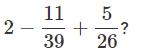Solution: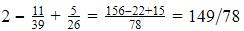QUESTION: 17

Which is the equivalent of -143/21

Solution:
QUESTION: 18

Of which property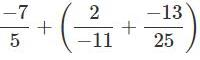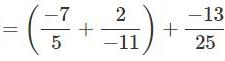is an example?

Solution:
QUESTION: 19

Which of the following statements is correct?

Solution:

All the given statements are correct. Consider 4/5 [a]
Since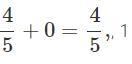is the additive identity of rational numbers. [b]
Since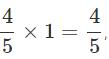is the  multiplicative identity of rational numbers. [c]
Since 0+0 = 0,0 is the additive inverse of 0.

QUESTION: 20

The sum of two rational numbers is −3. If one of the numbers is −7/5, find the other number.

Solution:

Let x be the required number. So,

x+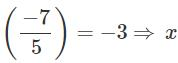=−3+ 7/5